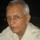Coffee Room
Discuss anything here - everything that you wish to discuss with fellow engineers.
12933 Members
Join this group to post and comment.Aruwin • Apr 18, 2012

conversion of base numbers

Somebody please help me with this. I need explanations and working.

85(base 10) 85/8=10.......5
10/8=1.........2
1/8 =0.........1

Why does the coversion of base numbers can be correctly calculated by the method above?Prove it.Jyothi Jo • Apr 19, 2012
Aruwin
Somebody please help me with this. I need explanations and working.

85(base 10) 85/8=10.......5
10/8=1.........2
1/8 =0.........1

Why does the coversion of base numbers can be correctly calculated by the method above?Prove it.

I didn't found any proof for this but logically it can be presented , for example if u take the decimal number 85 its binary equivalent is 1010101 converting to octal is nothing but grouping three bits and usually its answer is 125 but for large numbers it is quite difficult to convert to binary and group each three bits so the above method is used.Basically base conversions are used for the ease of the operator who operates computer because computer can understand only binary bits we need conversions to understand . For binary equivalent we use 0 and 1 and for octal equivalent we use 0 to 7 .So to check the binary equivalent of 85 we divide it by 2 and remainder whether it is 0 or 1 is noted from LSD to MSD (remainders )

similarly in the case division by octal,we divide by 8 and find the remainders from 0 to 7 and then convert them to binary .In octa and hexa, arithmetic operations are not done directly first they are converted to binary and are done.
Hence to convert a decimal integer to octal ,successively divide the given decimal number by 8 till the quotient is zero. The last remainder is MSB . The remainders read from bottom to top to give octa-equivalent. For brief understanding refer Switching Theory and Logic Design-by Anandkumar, A.P Godse,ABCD ABCD • Apr 19, 2012
consider converting decimal to binary: 6 to binary

2|6...0
2|3...1
1
So the method you've used above is similar to what you use to convert from decimal to octal. I Hope this helps.Aruwin
Somebody please help me with this. I need explanations and working.

85(base 10) 85/8=10.......5
10/8=1.........2
1/8 =0.........1